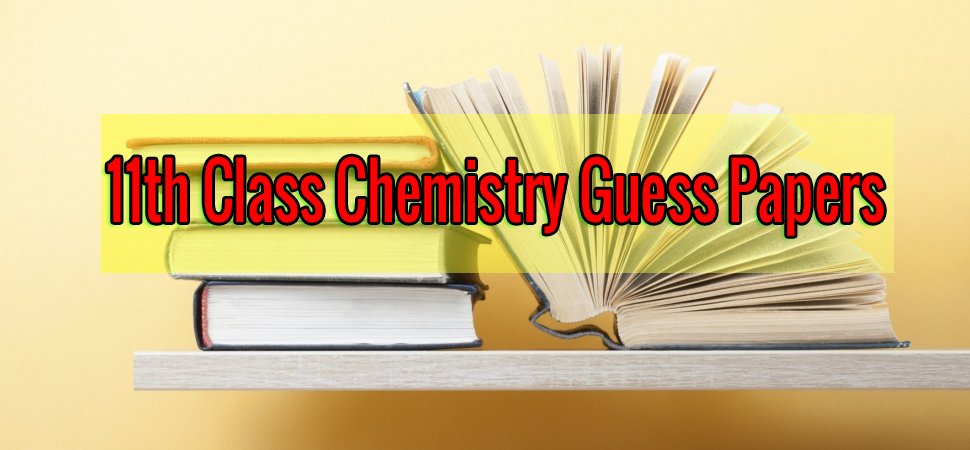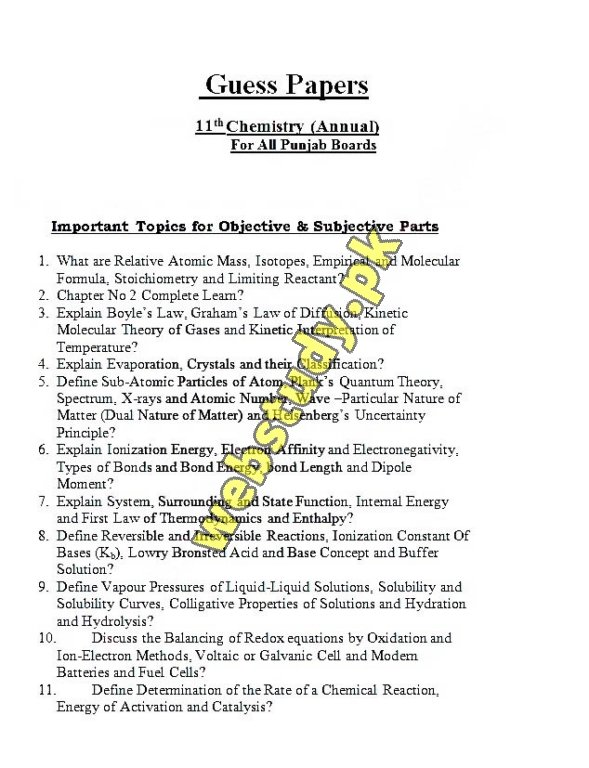# Inter 1st Year Chemistry Guess Papers 2019Chemistry is one of the important subjects in Intermediate and students looks worried about this subject. There are many reasons behind this as this subject is in English languages and most of the students studies Urdu medium in matric so in result they failed to get the idea of the chapter and hence they can not gain good marks in Chemistry. Most of the students search on internet for 11th Class Chemistry Guess papers 2019 so that they can prepare some paper and can get passing marks. Here at webstudy.pk all the students of intermediate who are having Chemistry as a subject can download free 11th Class Chemistry Guess Papers 2019 Online. All the students of intermediate ie F.Sc, Icom, ICS can download it and can prepare their examinations.

## 11th Class Chemistry Guess Papers 2019

Every  year thousands of students give examination of Intermediate Part 1 and most of the students can not pass the chemistry subject so for those weak in study students 1st Year Chemistry Guess Papers 2019 are posted here that they can download in pdf file online free. As most of the students have smartphone and laptop so now this is very easy for everyone to download chemistry guess paper 11th class 2019. Here in below you can read all the 11th Class Chemistry Important Questions 2019 Examinations. Important Short questions of chemistry 11th, objective and subjective model papers are also available to download here for free.

### Inter Part 1 Chemistry Guess Paper 2019

1. Give postulates of Bohr’s atomic model. Also describe defects of Bohr’s atomic model.  Give two statements of Raoult’s law. Derive the equation for the radius for nth orbit of hydrogen atom using Bohr’s model. State different forms of Raoult’s law. Also differentiate b/w ideal and non-deal solutions.
2. What is order of reaction? Explain various methods for finding order of reaction. Describe Arrhenius equation. Explain the effect of temperature on the rate of reaction with the help of Arrhenius Equation. Describe how the rate of a reaction can be measured by a chemical method? Also describe how Arrhenius equation helps us to calculate the energy of activation of a reaction.
3. Give graphical explanation for elevation of boiling point of a solution by Landsberger’s Method and derive an equation. What is the difference b/w single electrode potential and standard electrode potential? What is the effect of the boiling point on external pressure? Why the temperature of liquid remains at boiling point although heat is continuously supplied.
4. Explain the measurement of electrode potential with the help of Standard Hydrogen Electrode (SHE). Also describe the construction of voltaic cell and reaction occurring in the cell. Describe Dalton’s law of partial pressure. Write its applications. Define vapour pressure. Describe the measurement of vapour pressure by monometric method.
5. Describe Milikan’s oil drop method for determination of charge of electron. State and explain first law of thermodynamics. OR Prove that H=qv. How the charge on electron was measured by oil drop method?
6. What is orbital/atomic hybridization? Give its advantages Discuss SP2 hybridization with example. Explain bonding and structure of ethene (CH) on the basis of hybridization? Classify solids on the basis of binding. Define ionic solids. How ionic solids are formed? Give properties of ionic solids? Explain the geometry of NH3 and H20 by hybridization.
7. Write main postulates of VSEPR theory. Explain half life method for determining order of reaction. Give salient features of this theory. Also explain the structure of NH3 and H20 on the basis of this theory.
8. State and explain with an example, the Hess’s law of constant heat summation. Explain Enthalpy and prove that f= qe.
9. The solubility of PbF2 at 25c. Calculate the pH of buffer solution in which.
10. Differentiate b/w homogeneous & heterogeneous catalyst with examples. Give the basis of the molecular orbital theory and explain the paramagnetic character of O2 molecule on the basis of Molecular Orbital Theory (M.O.T)?
11. State and explain Graham’s law of diffusion from kinetic molecular theory of gases. Also give its experimental verification. What are molecular solids? Give their properties.
12. Benzoic acid, C6H5COOH, is a week mono baric acid. Write a note on lead accumulator with its discharge and recharging.
13. What are enzymes? Explain how do they act as catalysts? Also give characteristics of enzyme catalyst NHS can be produced by heating together.
14. Mg reacts with HCI to give hydrogen gas. What is a galvanic cell? Give its composition & working of galvanic cell.
15. What is an ideal gas? Real gases deviate more from ideal behavior at low temperature and under high pressure, explain. Write a note on construction and working of bomb calorimeter. Describe bomb-calorimeter method for determination of enthalpy of reaction.
16. Differentiate b/w isomorphism & polymorphism. Describe the electrolysis of molten sodium chloride & concentrated solution of sodium chloride.
17. What are cathode rays? How these rays are produced? Write their properties. Define solubility. Explain solubility curve.
18. What is mass spectrometer? How is it used to determine the relative atomic masses of isotopes? How are positive rays produced in discharge tube? Give properties of these rays 2014, 2015.
19. Write a note on catalysis. Distinguish b/w homogeneous and heterogeneous catalysis with suitable examples. Write characteristics of the catalyst. Also discuss types of catalyst.
20. Define yield. How do we calculate the percentage Vield of a chemical reaction? What is electrochemical series? Give its any three applications. Diferentiate b/w actual and theoretical yield. Why actual yield is less than theoretical yield?
21. What are azeotropic mixtures? What type of deviation is shown by them? Explain with the help of graph. What are liquid crystals? Give their three uses.